# Preface

This section discusses nonlinear differential equation used to model vibration of springs,

# Nonlinear Equations

When we try to describe the world around us and ourselves, it turns out that the corresponding models are inherently nonlinear. The simplest experiment illustrating this observation is an attempt to bend a plastic beam. As long as the load is small, the deflection of the beam is approximately follows Hooke's law. But at some sufficiently large level the beam will simply deform or break. This strong and definitely irreversible change is an elementary example of nonlinear behavior illustrates an important feature enforcing us to formulate the first statement more precisely. The world is nonlinear, however, in many cases, if we consider only small influences and changes, the linear approximation is often sufficient to understand, predict, and control its behavior.

Example: In eighteen century, Herman von Helmholtz was aware that human's ear perceived frequencies that are not contained in the incident acoustic radiation. To understand this phenomenon, let us treat the eardrum's tympanic membrane as a mechanical system system undergoing one-dimensional vibrations about its equilibrium position, the displace ment being denoted by y(t). Applying Nwton's second law, we can write the restoring force for small y as the Taylor expansion
$F(y) = F_0 + \left( \frac{{\text d}F}{{\text d}y} \right)_{y=0} y + \frac{1}{2} \left( \frac{{\text d}^2 F}{{\text d}y^2} \right)_{y=0} y^2 + \frac{1}{3!} \left( \frac{{\text d}^3 F}{{\text d}y^3} \right)_{y=0} y^3 + \cdots .$
In equilibrium, y = 0, the restoring force F(y) must vanish, so F0 = 0. Keeping two terms in the above expansion, we get
$F(y) = \left( \frac{{\text d}F}{{\text d}y} \right)_{y=0} y + \frac{1}{2} \left( \frac{{\text d}^2 F}{{\text d}y^2} \right)_{y=0} y^2 .$
To be a restoring force, the first coefficient $$k= -\left( {\text d}F/{\text d}y \right)_{y=0}$$ <\span> must be negative for positive y. The second coefficient of y² we denote by -ε, which gives us
$F(y) = -k\,y -\varepsilon\,y^2$
that is valid for small y.

Suppose that an eardrum is being driven by a time(t)-dependent sinusoidal pressure wave. Suitably normalized and with arbitrary parameter values, the nonlinear ODE for the eardrum displacement y(t) from equilibrium is of the form

$\ddot{y} (t) + y(t) +0.1\,y^2 (t) = 0.25\,\sin \left( 0.15\,t \right) ,$
subject to the initial conditions y(0) = 2 and $$\dot{y}(0) =-0.1 .$$ We solve the given initial value problem and plot the solution with the following Mathematica code
ode = y''[t] + y[t] + 0.1*(y[t])^2 == 0.25*Sin[0.15*t]
sol = NDSolve[{ode, y == 2, y' == -0.1}, y, {t, 200, 300}, MaxSteps -> 5000, Method -> "ExplicitRungeKutta", AccuracyGoal -> 10, PrecisionGoal -> 10]
Plot[Evaluate[y[t] /. sol], {t, 200, 300}, PlotPoints -> 1000, Frame -> True, PlotStyle -> Hue[0.9], FrameLabel -> {"time", "displacement"}, ImageSize -> {600, 400}, FrameTicks -> {{350, 500}, {-2, 0, 2}, {}, {}}, LabelStyle -> {FontFamily -> "Times", FontSize -> 16}]
However, when we increase the amplitude of driven force, the solution becomes unbounded.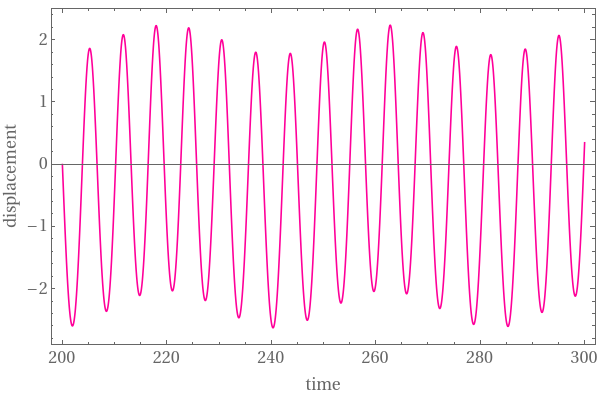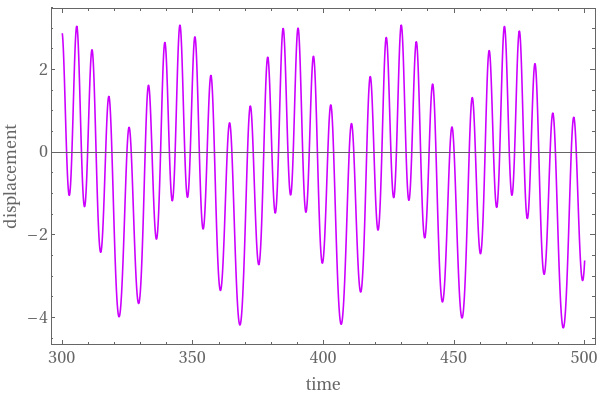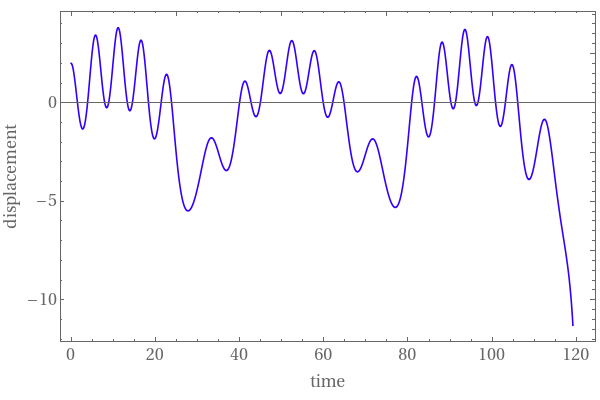Graph of the solution under the force $$0.25\,\sin \left( 0.15\,t \right)$$ Graph of the solution under the force $$1.25\,\sin \left( 0.15\,t \right)$$ Graph of the solution under the force $$2.25\,\sin \left( 0.15\,t \right)$$
We can also plot the velocity versus the displacement.
ParametricPlot[ Evaluate[{y[t], y'[t]} /. sol, {t, 0, 122}, Frame -> True, PlotStyle -> Hue[0.9], ImageSize -> {600, 400}, FrameTicks -> {{-2, -1, 0, {0.75, "y"}}, 1.5, {-1, 0, {0.5, "v"}, 1}, {}, {}}]]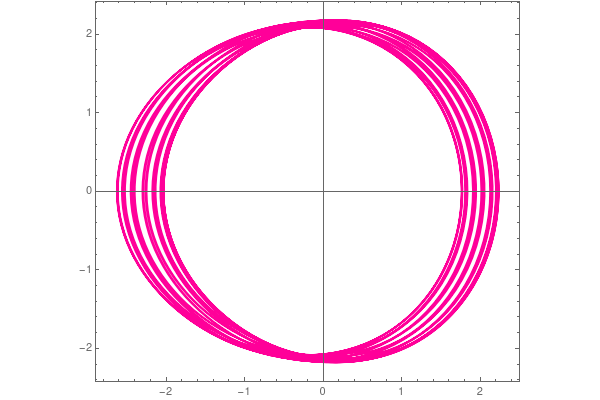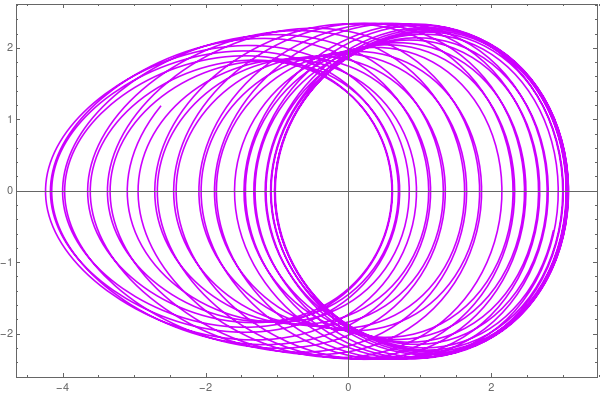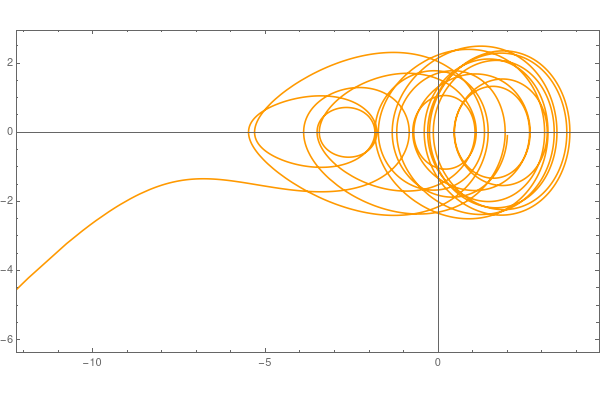Parametric plot v vs y under the force $$0.25\,\sin \left( 0.15\,t \right)$$ Parametric plot v vs y under the force $$1.25\,\sin \left( 0.15\,t \right)$$ Parametric plot v vs y under the force $$2.25\,\sin \left( 0.15\,t \right)$$
■

1. Nayfeh, A.H. and Mook, D.T., Nonlinear Oscillations, Wiley, New York, 1979.